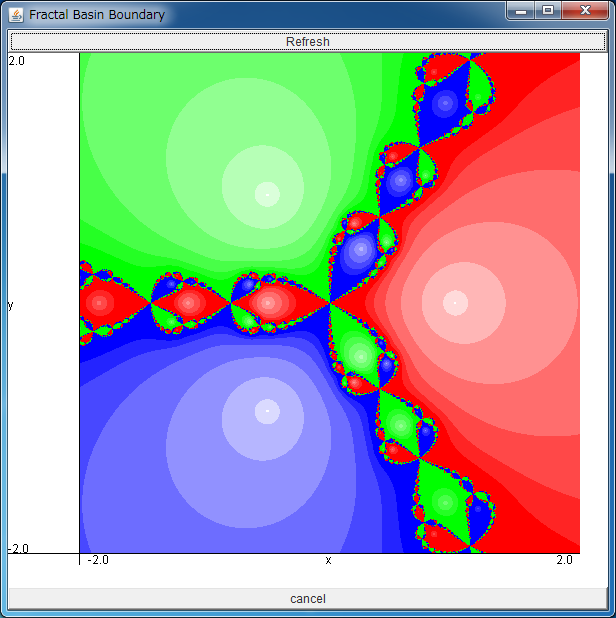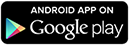# Fractal Basin BoundaryAfter downloading newton.jar, please execute it by double-clicking, or typing "java -jar newton.jar".

You can expand the area by dragging the field with your mouse.

If the above application does not start, please install OpenJDK from adoptopenjdk.net.

Android app of this application is also available. >>Let us consider an equation of complex number
z 3 = 1.
It can solved analytically and its solutions are z=1, exp(i2 π/3), and exp(i4 π/3).

The Newton method is one of the methods to solve such equations numerically.
For an equation f(z)=0, the Newton method tells us that the sequence
zn+1 = zn - f(zn)/ f'(zn)
converges to the true solution if the initial condition z0 is sufficiently close to it.

Then, let us return to the equation f(z) = z3-1 =0 and consider the initial conditions which converge to the true solutions 1, exp(i2 π/3), and exp(i4 π/3).
How do they distribute on the complex plane, and what geometrical properties do their boundaries have?
You can answer the above questions using the simulator in this page. The true solutions (x,y) = (1, 0), (-1/2, √3/2) and (-1/2, -√3/2) are shown at the centers of the three white circles, and the initial points which converge to the true solutions are colored with red, green, and blue, respectively. The whiter color means the rapid convergence to the solution.

As you see, the boundaries of three regions have very complex structures.
By expanding the area, you will notice that the boundaries have fractal structures,
and its complexity does not disappear no matter how largely you expand the field.

Generally, the set of the initial conditions which converge to an attractor is called its basin,
and the boundary with fractal structures as shown in the above simulator is called fractal basin boundary.

Though the above model is a mathematical one, it is known that the fractal basin boundary can be observed even in the real physical system.
When the system has several stable states, and the initial condition is placed nearby the fractal basin boundary, it means that one needs the information about the initial condition with the infinitely high precision to predict the final state of the system.
Note that this fact has given a large impact on physicists.

Moreover, when the attractors are chaotic, it is known that the basins can be intermingled almost everywhere in the phase space. Such basins are called riddled basin, and you can also simulate it in "Riddled Basin" simulator.

By the way, there also exists "periodic orbits" and "chaotic orbits" which do not converge to the true solutions on the basin boundaries in this simulator.
However, they are not attractors and their Lebesgue measures are 0, thus you can not observe them no matter how largely you expand the field.